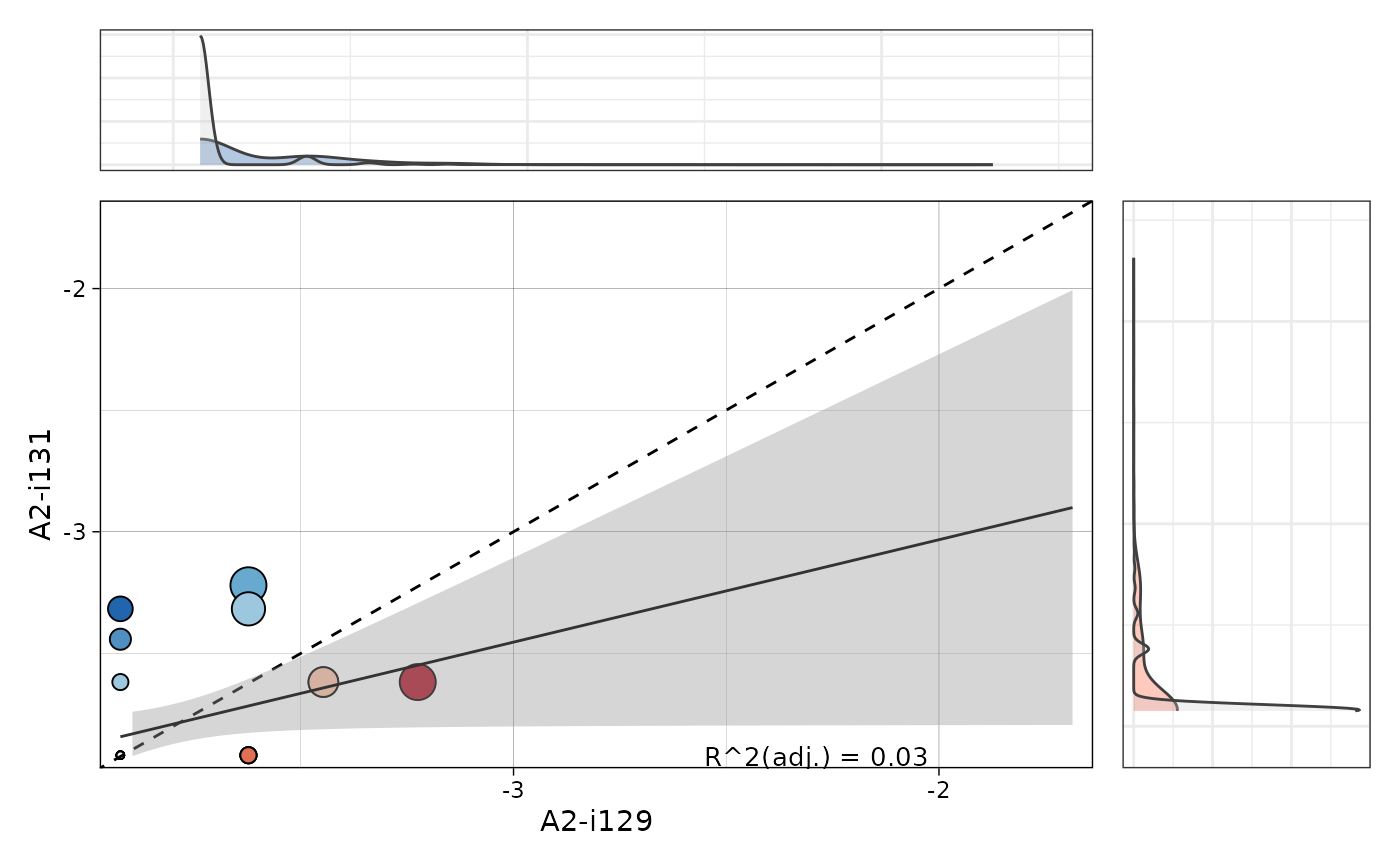Visualise correlation of public clonotype frequencies in pairs of repertoires.

vis_public_clonotypes(
.data,
.x.rep = NA,
.y.rep = NA,
.title = NA,
.ncol = 3,
.point.size.modif = 1,
.cut.axes = TRUE,
.density = TRUE,
.lm = TRUE,
)

## Arguments

.data

Public repertoire data - an output from the pubRep function.

.x.rep

Either indices of samples or character vector of sample names for the x-axis. Must be of the same length as ".y.rep".

.y.rep

Either indices of samples or character vector of sample names for the y-axis. Must be of the same length as ".x.rep".

.title

The text for the title of the plot.

.ncol

An integer number of columns to print in the grid of pairs of repertoires.

.point.size.modif

An integer value that is a modifier of the point size. The larger the number, the larger the points.

.cut.axes

If TRUE then axes limits become shorter.

.density

If TRUE then displays density plot for distributions of clonotypes for each sample. If FALSE then removes density plot from the visualisation.

.lm

If TRUE then fit a linear model and displays an R adjusted coefficient that shows how similar samples are in terms of shared clonotypes.

An integer value, that defines the size of the The text for the R adjusted coefficient.

## Value

A ggplot2 object.

data(immdata)
#> geom_smooth() using formula = 'y ~ x'